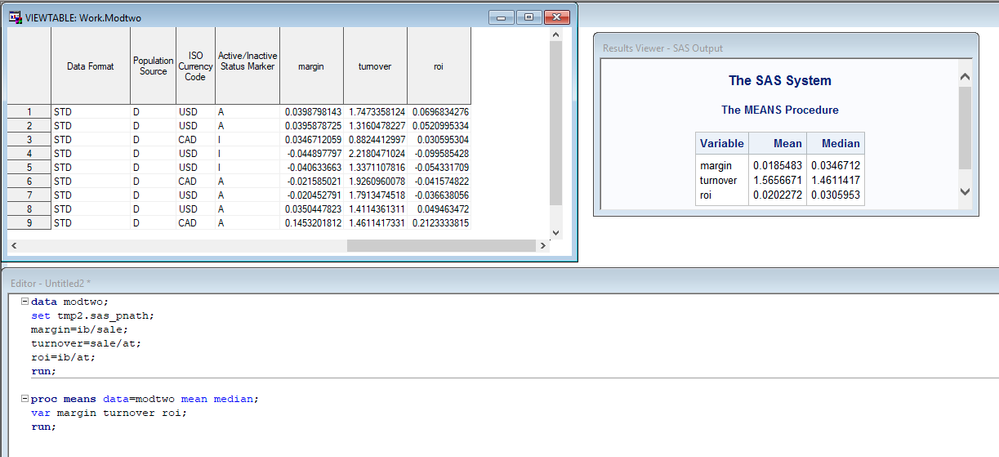## How do I create a new variable using an if then statement for above median and below median value?

How do I create a new variable using an if then statement for above median and below median roi value? I want to create a new column using data syntax, that will show value 1 for ROIs above median roi, otherwise 0.

So far I got to this point below:5 REPLIES 5

## Re: How do I create a new variable using an if then statement for above median and below median valu

Hi,

Welcome to the Communities forum.

If I've understood your requirements correctly, does the following help (essentially the last step creates the new column, the rest is just set up):

``````/* set up sample roi data */
data have;
input roi;

datalines;
1
2
3
4
5
;

/* set up median roi value */
%let median_roi = 3;

data want;
set have;

/* use greater than comparison operator to assign 1 (true) or 0 (false) */
new_column = roi gt &median_roi;
run;
``````

If you need something different, then please show what results you are looking for for each row and confirm the logic behind it.

Thanks & kind regards,

Amir.

## Re: How do I create a new variable using an if then statement for above median and below median valu

Use PROC RANK and 2 ranks to split the data?

## Re: How do I create a new variable using an if then statement for above median and below median valu

``````proc rank data=modtwo out=want groups=2 ties=low;
var roi;
ranks rankROI;
run;``````

@prn21005 wrote:

How do I create a new variable using an if then statement for above median and below median roi value? I want to create a new column using data syntax, that will show value 1 for ROIs above median roi, otherwise 0.

So far I got to this point below:## Re: How do I create a new variable using an if then statement for above median and below median valu

First, realize that you will eventually need to deal with realistic questions.  What should happen with ROI:

• Is exactly equal to the median?
• Has a missing value?

Here is an approach that extends tools that you already know, to get the result:

``````proc summary data=have;
var roi;
output out=medians (keep=roi_median) median=roi_median;
run;``````

That gives you the median in a data set, which can be used in a subsequent step.:

``````data want;
set have;
if _n_=1 then set medians;
roi_group = roi >= roi_median;
drop roi_median;run;``````

Note that the logic can easily be expanded to compute/group for more than one variable, without increasing the number of steps.  For example, you could begin with:

``````proc summary data=have;
var roi margin turnover;
output out=medians (keep=roi_median margin_median turnover_median) median=roi_median margin_median turnover_median;
run;``````

Also note that the assignment statement that computes roi_group could have been accomplished with IF/THEN statements, but would take more code:

``````if roi >= roi_median then roi_group=1;
else roi_group = 0;``````

But the assignment statement shown above accomplishes the same with a shorter program.

## How do I create a new variable using an if then statement for above median and below median value?

Discussion stats
• 5 replies
• 378 views
• 2 likes
• 5 in conversation